### Home

#### Numerical Algorithms and Tensor Learning Laboratory

Numerical Algorithms and Tensor Learning Laboratory

#### Dr. Ratikanta Behera

Welcome to the Numerical Algorithms and Tensor Learning Lab at the Indian Institute of Science, Bangalore. We are a part of Department of Computational and Data Sciences (CDS). The research lab is established in May 2022 with Ratikanta Behera as the convenor.

We are developing adaptive recurrent neural networks to solve time-varying tensor equations. Our goal is to design innovative, scalable, and efficient tensor-based algorithms supported by theoretical principles to solve significant existing and emerging multidimensional problems. We are also proposing HPC-centric adaptive wavelet algorithms for solving PDEs, integral equations, and signal & image processing.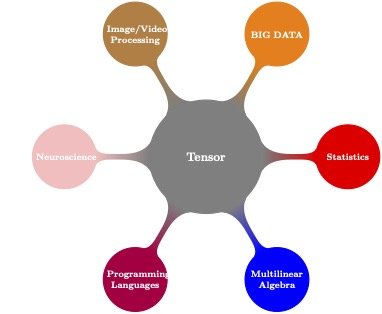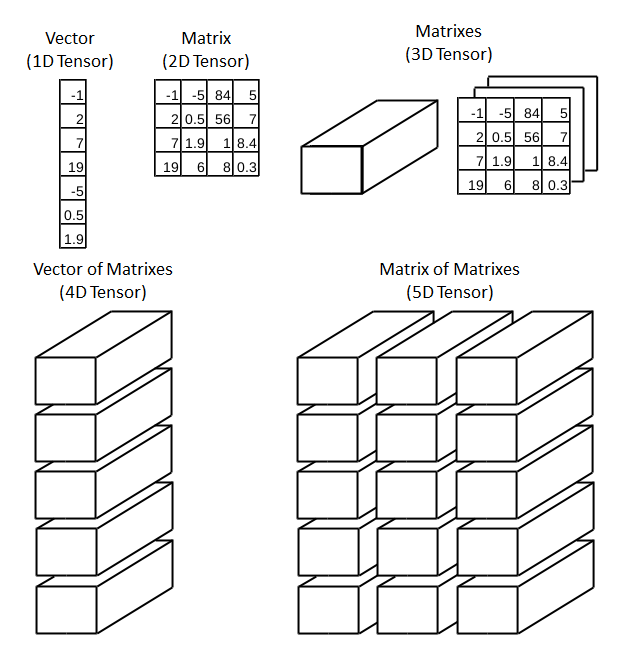In the era of BIG data, artificial intelligence, and machine learning, we are faced with the need to process multiway (tensor-shaped) data. These data are mostly in the three or higher-order dimensions, whose orders of magnitude can reach billions. Huge volumes of multi-dimensional data are a great challenge for processing and analyzing; the matrix representation of data analysis is not enough to represent all the information content of the multiway data in different fields. A tensor is a higher-dimensional generalization of a matrix (i.e., a first-order tensor is a vector, a second-order tensor is a matrix) has a core of many applications in diverse scientific computing and data science domains. However, tensor computations present several challenges due to their complexity, high computational cost, and large memory footprint. We develop novel HPC-driven algorithms and theories to deal with the most computation-intensive aspect of engineering and scientific applications. Specifically, interested in developing fast tensor algorithms for solutions to multilinear systems, nonlinear optimization problems, low-rank approximation, generalized inverses of tensors, and solutions to partial differential equations in high dimensions problems. Further, we design adaptive recurrent tensor neural networks to solve practical engineering applications (i.e., output tracking control problems, current flows in an electrical network, computation of the Wheatstone bridge, etc.) In particular, we aim to prove theoretically and numerically the behavior of adaptive recurrent tensor neural networks under various activation functions.Mathematical modeling of problems in science and engineering typically involves solving partial differential equations. However, in many situations, the small spatial scales are highly localized, and thus the efficient and accurate solution of the problem requires a locally adapted grid. Traditional adaptive algorithms are costly because grids can change drastically within a short time interval. Thus, conventional algorithms on a uniform grid are inefficient for astrophysics, material sciences, meteorology, combustion problems, and turbulence modeling. We aim to develop a dynamically adaptive wavelet collocation algorithm to deal with problems with localized structures, which might occur intermittently anywhere in the computational domain or change their locations and scales in space and time. We propose HPC-centric adaptive wavelet algorithms for solving partial differential equations and integral equations, data compression, signal recognition, and signal & image processing.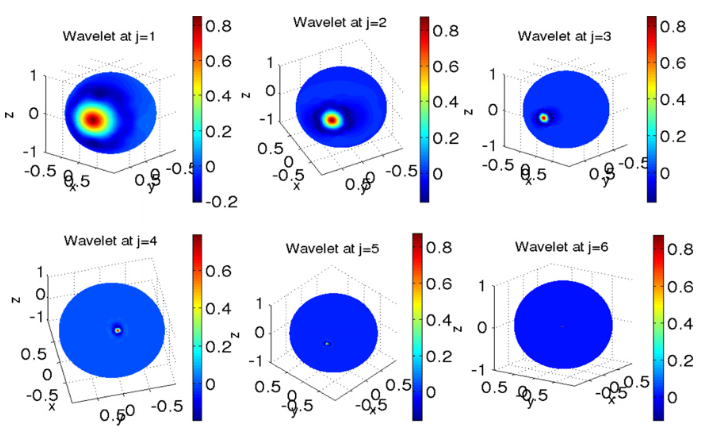We present the multilevel adaptive wavelet collocation method to solve a convection-dominant problem over the spherical geodesic grid. This method is based on a multi-dimensional second-generation wavelet over a spherical geodesic grid. The method is more helpful in capturing, identifying, and analyzing local structure than any other traditional methods (i.e., finite difference, spectral method). For more details please see here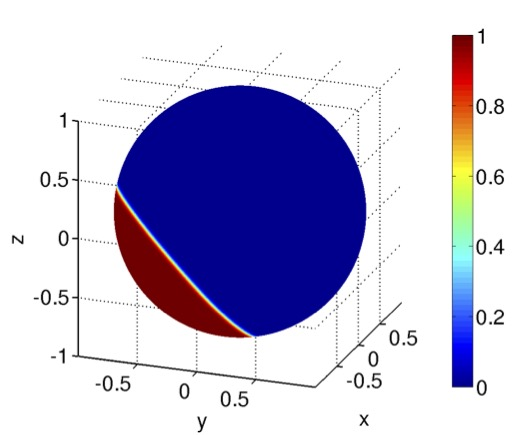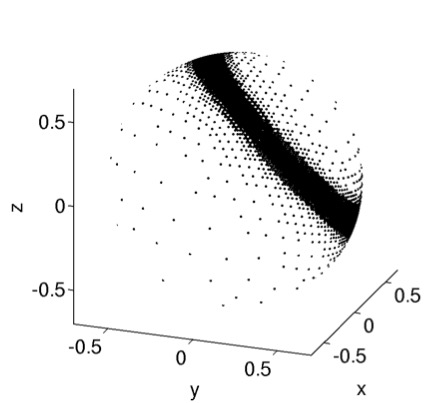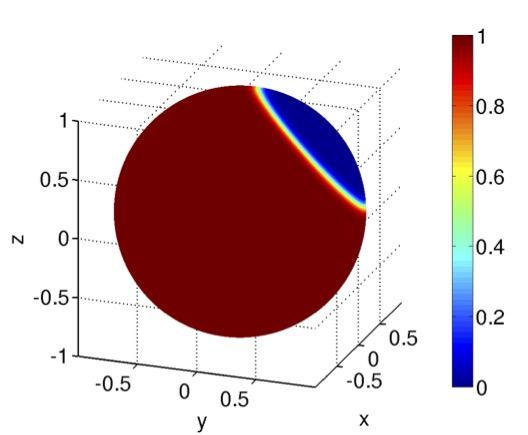Our lab accommodates the studies undertaken on the topics related to but not limited to:

• High-performance tensor contractions in scientific computing

• Tensor generalized inverses and applications

• Tensor decompositions and approximations

• High-performance algorithms and implementations of tensor operations

• Optimization of algorithms for tensor computations

• Adaptive space-time wavelet algorithm for PDEs

• Design adaptive space-time curvelets and its applications

• Dynamic adaptive algorithm for image encryption

• Tensor methods in applied computational domains

• Tensor operations with application to Machine and Deep learning